# Decimals

## What are Decimal Numbers?

A fraction is a part of a whole. The whole itself can be a number, an object or a group of numbers (or objects). Fractions help us speak of numbers besides whole numbers. For instance, 15 and 16 are whole numbers — but a fraction is needed to write out a number that is between 15 and 16. Decimals help us like fractions do — but are separate entities.

This number line shows us a few whole numbers on the number line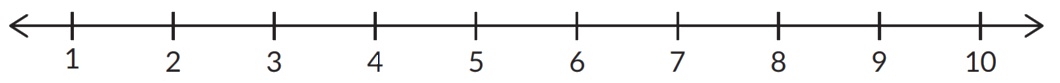Fractions and decimals both exist on the number line. In fact, every fraction has an equivalent decimal. The real difference between the two is how they are written out.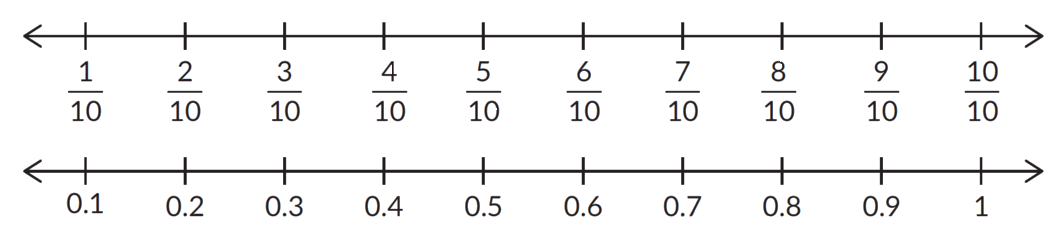## The place value system

We know that the whole number 315 can be written out as 300 + 10 + 5. Here's how the number looks when it's put within a place-value chart.

 Hundreds Tens Units 3 1 5

And here is how 316 looks.

 Hundreds Tens Units 3 1 6

But what about a number that is between 315 and 316? 3152/10 is a fraction that lies between the two numbers. What is this number in decimal-notation?

The denominator 10 reappears as a point in the decimal number 315.2

Here's how this decimal number can be put into a place-value chart.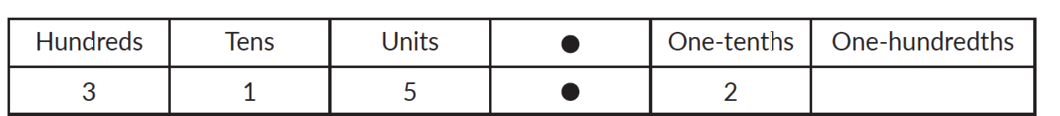We've learnt that the place-names of the digits in a whole number are ones, tens, hundreds, thousands etc. This holds for decimal numbers too — up to the decimal point. The digits after the decimal point have the place-names tenths, hundredths, thousandths etc. (Look at the chart given above.)

Note: In a decimal number, every digit before the point is a whole number and every digit after the point is a part of one whole. Consequently, the place-value of a digit after the point is always less than 1.

We can use a model to better understand decimal numbers. The flat, rod and block model (or the FRB model) is a convenient one.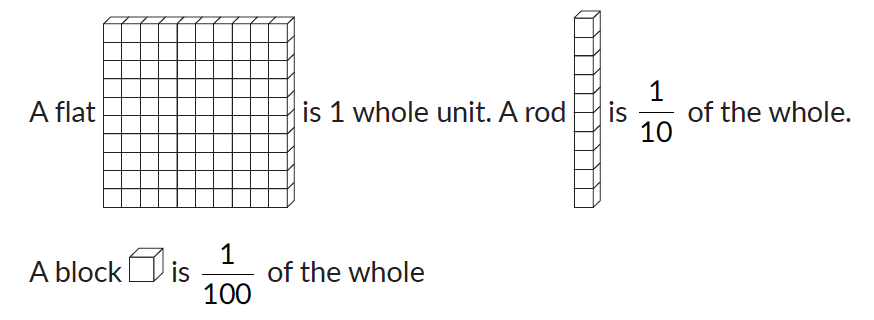The number 1.26 can be represented by 1 flat and 26 blocks. Which is the same as 1 flat, 2 rods and 6 blocks.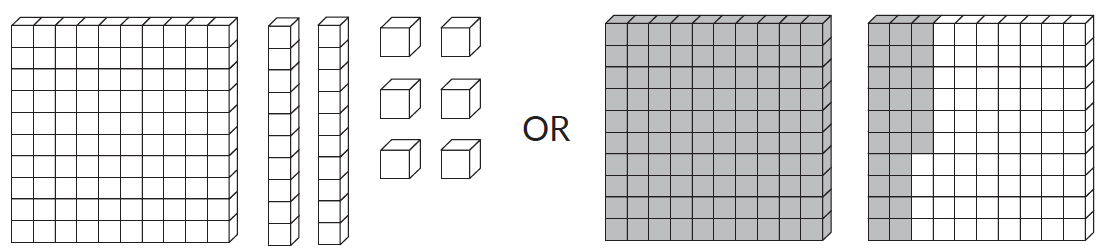## Here is how Cuemath students learn to visualise decimal numbers

Experience this in a Cuemath class. Book a Cuemath demo class with a nearby centre.

## Visualisation and Comparison of Decimals

Given below is a video to understand how to visualise decimal numbers using flats, rods and blocks. Children often get confused with comparison of decimal numbers. This video is to see how easily can the decimal numbers be compared.

## Topics closely related to Decimals

The chart shown below shows all the topics that are pre-requisite to understanding Decimals. Also, all those topics which require a fair understanding of Decimals.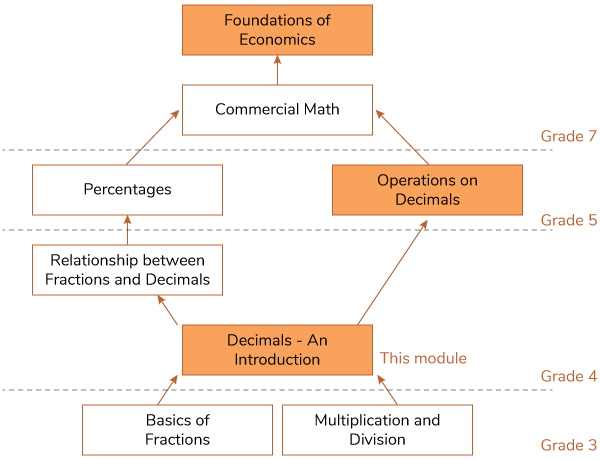## Why are decimal numbers important?

Many times, children ask, “What’s the point of decimals?”

Firstly, as decimals allow us to express numbers between two whole numbers, they allow us to make precise measurements. The more the decimal places, the greater the precision.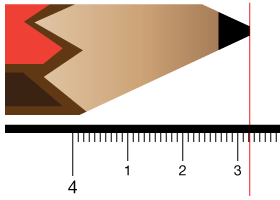Secondly, they allow us to express fractions in a manner that lets us use place values on a number line for easier arrangement and more accuracy.

## How Do you Teach Decimals?

1. Help students visualise it using different models like abacus, flats, rods and blocks.

2. Have a bunch of flashcards with decimal numbers written on it. You can ask your child to read as many as possible in 30 seconds.

3. Have a bunch of flashcards with decimal numbers written on it and a digit underlined. You can ask your child the place name of that digit.

## FAQ

Q1. What is the difference between a decimal & a whole number?

Whole numbers specify how many elements there are in a collection of objects while decimals are a part of the whole numbers.

Q2. What decimal of an hour is a second?

\begin{align}={1 \over {60 \times 60}}\end{align}

\begin{align}= {1\over 3600}\end{align}

$$= 0.00027$$

## Important Topics

Here are a few links that will take you through the journey that every Cuemath students undertake in the pursuit of understanding Decimals along with practice worksheets:

More Important Topics
Numbers
Algebra
Geometry
Measurement
Money
Data
Trigonometry
Calculus
More Important Topics
Numbers
Algebra
Geometry
Measurement
Money
Data
Trigonometry
Calculus
Learn from the best math teachers and top your exams

• Live one on one classroom and doubt clearing
• Practice worksheets in and after class for conceptual clarity
• Personalized curriculum to keep up with school